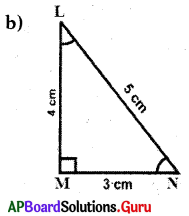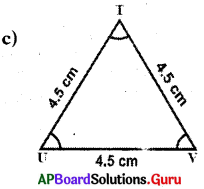# AP Board 7th Class Maths Solutions Chapter 5 Triangles Exercise 5.1

SCERT AP 7th Class Maths Solutions Pdf Chapter 5 Triangles Ex 5.1 Textbook Exercise Questions and Answers.

## AP State Syllabus 7th Class Maths Solutions 5th Lesson Triangles Exercise 5.1

Question 1.
Classify the following triangles based on the length of their sides.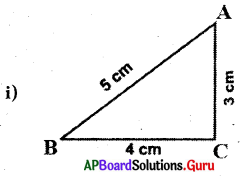In ∆ABC,
AB ≠ BC ≠ AC
So, ∆ABC is scalene traingle.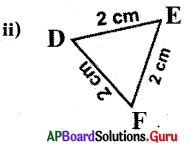In ∆DEF,
DE = EF = FD = 2 cm
So, ∆DEF is an equilateral triangle.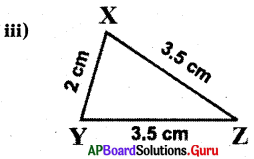In ∆XYZ,
XZ = YZ = 3.5 cm
So, ∆XYZ is an isosceles triangle.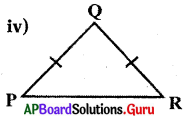Isosceles triangle (∵ Two sides are equal)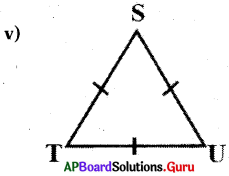Equilateral triangle (∵ Three sides are equal)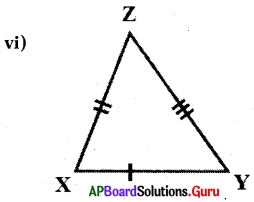Scalene triangle (∵ NO two sides are equal).Question 2.
Classify the following triangles based on the measure of angles.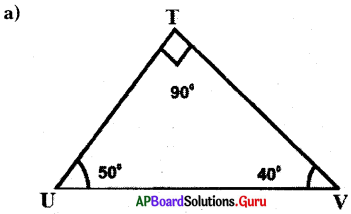In ∆TUV, ∠T = 90°
So, ∆TUV is Right-angled triangle.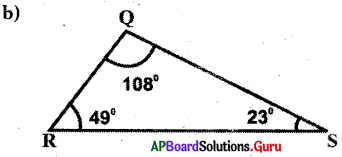In ∆QRS, ∠Q = 108°
So, ∆QRS is an Obtuse angled triangle.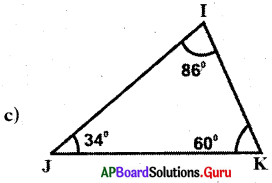In ∆IJK, all are acute angles.
So, ∆IJK is an acute angled triangle.

Question 3.
Classify the following triangles based on their sides and also on their angles.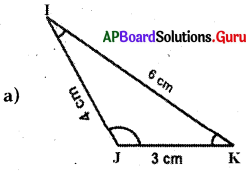In ∆IJK, IJ ≠ KJ ≠ IK
So, ∆IJK is a scalene triangle.